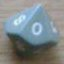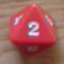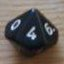Set Operators

## Set Operators

 Mon Sep 14 05:22:38 2015   by   wyrdR If it would not be too difficult please consider implementing built-in Set functions similar SubSet and EqualSet into Troll.  The it might be possible to run a command like:if A sub B then DoSomething(if A is a subset of B then do something)or accumulate x:=6d6 while {6,6} sub x(accumulate while there are two 6s in the roll)You can refer to user-contributed roll_ 'SubSet or EqualSet' for my fudged version of this.ThankswyrdR Mon Sep 14 08:31:22 2015   by   Torben If you are thinking of a multiset subset test, that is fairly easy to do: If A -- B is empty, then A is a multiset subset of B.  So to do something like "if A is a multiset subset of B then C else D", you would write "if A -- B then D else C".  Note the swapped branches of the if-then-else. as a function, you can define it by`function msubset(A,B) = if A--B then {} else 1`A "normal" subset test can be done similarly, but using the "drop" operator instead of --.Multiset equality can be found by mutual subset tests: If A is a (multiset) subset of B and B is a (multiset) subset of A, then A and B are the same (multi)sets.  Equivalently,`function msetEqual(A,B) = if (A--B) U (B--A) then {} else 1`I might add these as standard functions at some point, but since they are relatively easy to define, I will postpone until I want to do other changes too. Mon Sep 14 15:34:01 2015   by   wyrdR Thanks Torben,I was thinking that, but I was *over-thinking* it.Maybe just writing them up as examples in the next documentation will do :)wyrdR Tue Sep 15 11:16:00 2015   by   Torben I have added the functions as examples in the left pull-down menu.